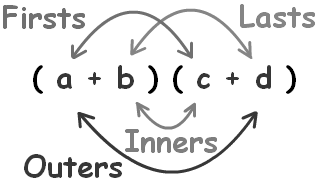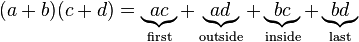# Foil Formula

FOIL is a mnemonic for the standard method of multiplying two binomials, hence the method may be referred to as the FOIL method.The word FOIL is an acronym for the four terms of the product:

• First (“first” terms of each binomial are multiplied together)
• Outer (“outside” terms are multiplied—that is, the first term of the first binomial and the second term of the second)
• Inner (“inside” terms are multiplied—second term of the first binomial and first term of the second)
• Last (“last” terms of each binomial are multiplied)

The general form is:### Solved Example

Question: Evaluate: (2x + 4)(5x + 3)

Solution:

Given expression is, (2x + 4) (5x + 3)

According to FOIL formula,

$$\begin{array}{l}(2x + 4) (5x + 3) = (2x \times 5x + 2x \times 3) + (4 \times 5x + 4 \times 3)\end{array}$$

$$\begin{array}{l}(2x + 4) (5x + 3) = 10x^{2} + 6x + 20x + 12\end{array}$$

$$\begin{array}{l}(2x + 4) (5x + 3) = 10x^{2} + 26x + 12\end{array}$$